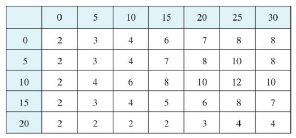Chapter 15.1, Problem 6E

Chapter
Section
Textbook Problem

A 20-ft-by-30-ft swimming pool is filled with water. The depth is measured at 5-ft intervals, starting at one corner of the pool, and the values are recorded in the table. Estimate the volume of water in the pool.To determine

To estimate: The volume of water in the swimming pool of dimension 20 ft×30 ft .

Explanation

Formula used:

The volume of water in the pool is, Vlimm,ni=1mj=1nf(xi,yj)ΔA ,

Where, ΔA=a2 , where a is the length of the side of the square.

The Riemann sum constants are m, n.

Given:

The surface, z=f(x,y) .

The rectangular region, R={(x,y)|0x20,0y30} .

Calculation:

Let the Riemann sum partitions be m=4,n=6 for the given rectangular region.

Plot the given rectangle in the graph and pick the sample points at the upper right corners of each square.

From Figure 1, it is observed that the upper right corners of each square is (5,5),(5,10),(5,15),(5,20),(5,25),(5,30),(10,5),(10,10),(10,15),(10,20),(10,25),(10,30,) (15,5),(15,10),(15,15),(15,20),(15,25),(15,30),(20,5),(20,10),(20,15),(20,20),(20,25),(20,30) and a=5 .

Therefore, ΔA=25 .

From the given table, it is observed that,

The value corresponds to x=5,y=5 is 3.

The value corresponds to x=5,y=10 is 4

Still sussing out bartleby?

Check out a sample textbook solution.

See a sample solution

The Solution to Your Study Problems

Bartleby provides explanations to thousands of textbook problems written by our experts, many with advanced degrees!

Get Started

Show that sin-1(tanh x) = tan1(sinh x).

Single Variable Calculus: Early Transcendentals, Volume I

Solve the equations in Exercises 126. x+4x+1+x+43x=0

Finite Mathematics and Applied Calculus (MindTap Course List)

In Exercises 4562, find the values of x that satisfy the inequality (inequalities). 50. 0 x + 2 4

Applied Calculus for the Managerial, Life, and Social Sciences: A Brief Approach

True or False: limx6x3+8x29x+75x34x+30=limx6x35x3.

Study Guide for Stewart's Single Variable Calculus: Early Transcendentals, 8th